# Neural Network Part1: Inside a Single Neuron

The perceptron or a single neuron is the fundamental building block of a neural network .The idea of a neuron is basic but essential .

Lets start understanding the forward propagation of information through a single neuron.

We define a sets of inputs to that neuron as x1 ,x2 …xn. And each of these inputs have a corresponding weight w1 …wn.What we can do is with each of these inputs and weights, we can multiply them correspondingly together and take a sum of all of them.We can take this summation which is a single number and pass it through what is called a non-linear activation function and that produces our final output y.Now this is not entirely correct,we also have a bias term added in this neuron .The purpose of bias is to actually shift your activation function to the left and to the right regardless of the inputs so bias is not affected by the x inputs it just that bias associates that input.

As you can see from the above diagram,the neuron diagram can be illustrated mathematically as a single equation.We can actually rewrite this equation using linear algebra using vectors and dot products .So instead of summation of all the x inputs and weights w i.e ΣXi*Wi ,we can actually change x into a vector of inputs X and we also have a vector of weights W.

To compute the output of a single perceptron ,we have to take the dot product of X and W which represents our multiplication and summation ΣXi*Wi and then apply non-linearity which is denoted as g.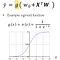Activation Function

So,what is this g or activation function ?So one example of activation function is the sigmoid activation function.The thing about sigmoid activation function is that it takes any real number as input on the X-axis and transforms it into a bounded output between 0 and 1 .Common example of sigmoid is when dealing with probabilities since probabilities are bounded between 0 and 1 and sigmoids are really useful when you want to output a single number and represent that number as a probability distribution.

In fact, there are many types of non-linear activation functions such as tanh,softmax,relu.So why do we need activation functions?

This is important because in real life almost all of our data is nonlinear.For example,if I told you separate green points from the blue points using a linear function ,you wont be able to produce good results,it would look something like this .No matter how deep or large a network is ,linear functions produce a linear output .You would be composing lines on top of lines and you are gonna get another line.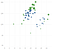Try to separate the blue and green points in this graph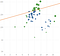Linear attempt to seperate the points

On the other hand ,non-linear activation functions or non-linearities allow you to approximate complex functions by introducing these non-linearities into your decision boundary.This is what makes neural networks extremely powerful.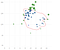Non-linear attempt

Now let’s take an example of of having bias w0=1 and W=[3,-2],so basically multiply our inputs with those weights and apply nonlinearity over it. But lets have a look at non-linearity before we apply it.So when we take the dot product of our inputs and weights ,the equation we get is a 2D line and we can plot this line if we equate this line to zero .

Now,if I feed a new input with co ordinates W=[-1,2] and apply this into the linear equation and generalize it we get -6 that is before we apply the non-linearity.And after we apply sigmoid activation function for -6 which is less than 0 we get a very low value 0.02 ,an output between 0 and 1.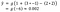After applying sigmoid activation function

We can actually generalize it, for any point on this plot ,we can tell where it will lie on the left side of the line that is before we apply non-linearity the state of that neuron will be negative less than zero and after applying non-linearity ,the sigmoid will give an output of less than 0.5 and on the right side of the line state will be zero and probability will be 0.5.

In conclusion ,remember how a single neuron works.You take the dot product of your weights and inputs ,add a bias and then apply non-linearity i.e that activation function on it to get the output.

This is the mechanism which happens inside a single neuron .Using this perceptron concept ,we can make multi output perceptron and going forward we can make a single layer neural network.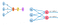Single Perceptron(left)Multi Output Perceptron (Right)Single Layer Neural Network

So that is all about a single neuron,next article will demonstrate about how neural networks work together or rather these perceptron come together to form a neural network.

## Analytics Vidhya

Analytics Vidhya is a community of Analytics and Data…

Written by

## Shweta Kadam

Software Engineer and always a Learner! https://shwetarkadam.github.io/portfolio

## Analytics Vidhya

Analytics Vidhya is a community of Analytics and Data Science professionals. We are building the next-gen data science ecosystem https://www.analyticsvidhya.com

Written by

## Shweta Kadam

Software Engineer and always a Learner! https://shwetarkadam.github.io/portfolio## Analytics Vidhya

Analytics Vidhya is a community of Analytics and Data Science professionals. We are building the next-gen data science ecosystem https://www.analyticsvidhya.com

## More From Medium

Medium is an open platform where 170 million readers come to find insightful and dynamic thinking. Here, expert and undiscovered voices alike dive into the heart of any topic and bring new ideas to the surface. Learn more

Follow the writers, publications, and topics that matter to you, and you’ll see them on your homepage and in your inbox. Explore

If you have a story to tell, knowledge to share, or a perspective to offer — welcome home. It’s easy and free to post your thinking on any topic. Write on Medium